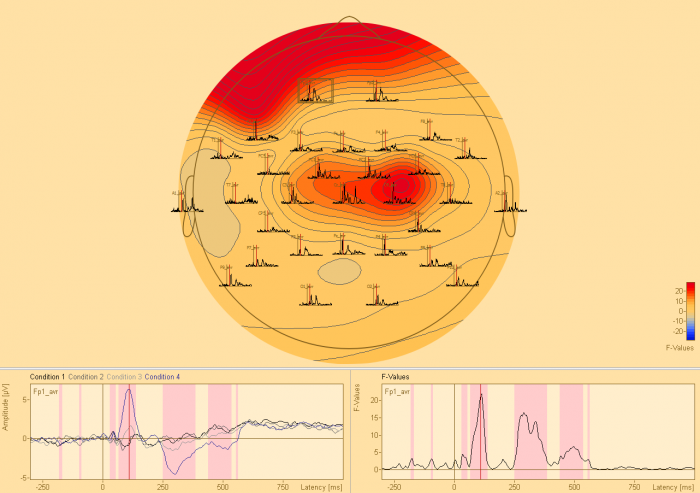# Preliminary Statistics

## Preliminary Statistics – The Input for Cluster-Permutation Testing

### t-test

Student’s t-test is computed to determine whether there is a significant difference between the mean of two groups / conditions.

A t-test can be paired or unpaired. A paired t-test is appropriate when there is an interdependency between the two conditions that are compared. This is generally the case if two conditions in the same set of subjects are compared. An unpaired t-test is appropriate when there is no interdependency between the two groups that are being compared. This is generally the case if two different groups of subjects are compared for the same condition.

### ANOVA / ANCOVA

A one-way analysis of variance ANOVA investigates the influence of an independent variable with two or more factor levels on a dependent variable. The null-hypothesis assumes that the means of all groups / conditions are equal. It gets rejected as soon as there are at least two groups / conditions with significantly different means, i.e. it is not required that all groups / conditions must differ from each other.

ANOVAs can be computed between-group or within-group (repeated measures). Between-group means that there is no interdependency between the factor levels of the independent variable. This is usually the case if different groups are compared. Within-group (repeated measures) means that there is a relationship between the factor levels of the independent variable. This is usually the case if different conditions within the same set of subjects are compared.

It is possible to add a covariate of no interest in the one-way ANOVA computation altering it to a one-way analysis of covariance (ANCOVA). “Covariate of no interest” denotes a factor that might influence the dependent variable in its own right, thus interacting with the independent variable of interest in an unwanted fashion.

### Correlation

Correlation analysis investigates the relationship between two variables. It is dependent on the covariance of the two variables, i.e. the estimate of how much the two variables are changing together. As an example, the relationship between blood cortisol level and EEG / MEG data can be investigated.

## Clustering based on the Preliminary Statistical Test

Clustering in time and if applicable space and frequency is based on the preliminary t-test, ANOVA / ANCOVA, or correlation. Depending on the cluster-alpha setting, adjacent data points will be subsumed to form a cluster. The probability of these data clusters will be determined during permutation testing.

### Preliminary Statistics• More that two groups / conditions can be compared (within / between-group ANOVA)
• A covariate of interest can be added to the analysis of variance (ANCOVA)
• Two groups / conditions can be compared with unpaired / paired t-tests

#### BESA Statistics

• Private: Features